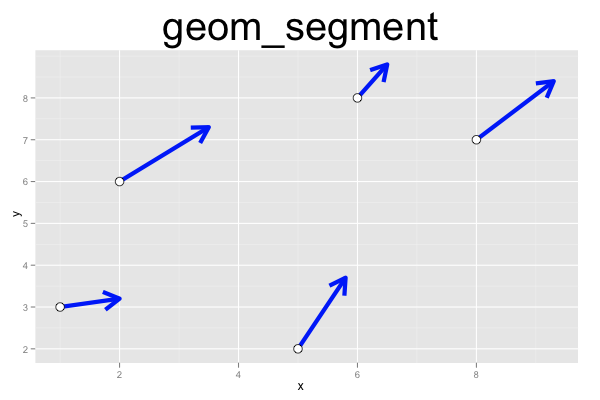## ggplot2 Quick Reference: geom_segment

A geom that draws a line segment defined by (x, y) and (xend, yend) coordinates.

Default statistic: stat_identity

### Parameters

• x - (required) x coordinate of starting point
• y - (required) y coordinate of starting point
• xend - (required) x coordinate of end point
• yend - (required) y coordinate of end point
• size - (default: 0.5) width of the line segment
• linetype - (default: 1=solid) the type of the line
• colour - (default: "black") the color of the line segment
• alpha - (default: 1=opaque) the transparency of the line segment
• arrow - (default: NULL) the arrow to draw at the end point of the line segment (use the `arrow()` function in R's `grid` package to create arrows)

### Example

This plot contains two layers. The bottom layer draws the line segments, with solid blue lines of width 2 ending in an arrow. The upper geom_point layer draws points at the starting points of the line segments (filled in white, with a black outline).```require(grid)
d=data.frame(x=c(1,2,5,6,8), y=c(3,6,2,8,7), vx=c(1,1.5,0.8,0.5,1.3), vy=c(0.2,1.3,1.7,0.8,1.4))
ggplot() +
geom_segment(data=d, mapping=aes(x=x, y=y, xend=x+vx, yend=y+vy), arrow=arrow(), size=2, color="blue") +
geom_point(data=d, mapping=aes(x=x, y=y), size=4, shape=21, fill="white") +
opts(title="geom_segment", plot.title=theme_text(size=40, vjust=1.5))```